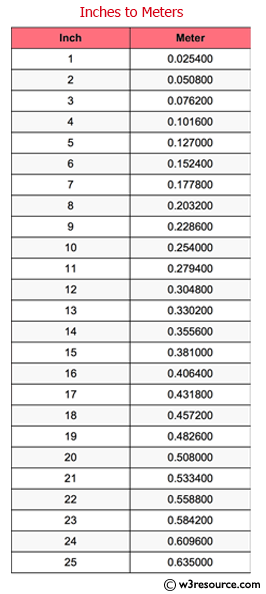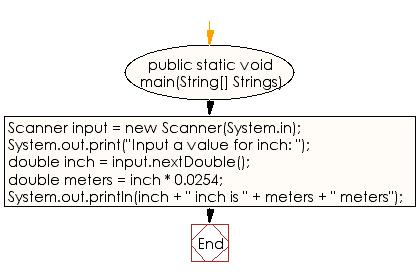﻿ Java exercises: Inches to meters - w3resource# Java Data Type Exercises: Inches to meters

## Java Data Type: Exercise-2 with Solution

Write a Java program that reads a number in inches, converts it to meters.

The inch is a unit of length in the (British) imperial and United States customary systems of measurement now formally equal to ​1/36 yard but usually understood as ​1/12 of a foot.

The meter is the base unit of length in some metric systems, including the International System of Units (SI). The SI unit symbol is m.

Note: One inch is 0.0254 meter.

Test Data
Input a value for inch: 1000Sample Solution:

Java Code:

``````import java.util.Scanner;
public class Exercise2 {

public static void main(String[] Strings) {

Scanner input = new Scanner(System.in);

System.out.print("Input a value for inch: ");
double inch = input.nextDouble();
double meters = inch * 0.0254;
System.out.println(inch + " inch is " + meters + " meters");

}
}
```
```

Sample Output:

```Input a value for inch: 1000
1000.0 inch is 25.4 meters
```

Flowchart:Java Code Editor:

Improve this sample solution and post your code through Disqus

What is the difficulty level of this exercise?

﻿

## Java: Tips of the Day

Checks if a string is upper case:

```public static boolean isUpperCase(String input) {
return Objects.equals(input, input.toUpperCase());
}
```

Ref: https://bit.ly/39Hpo84